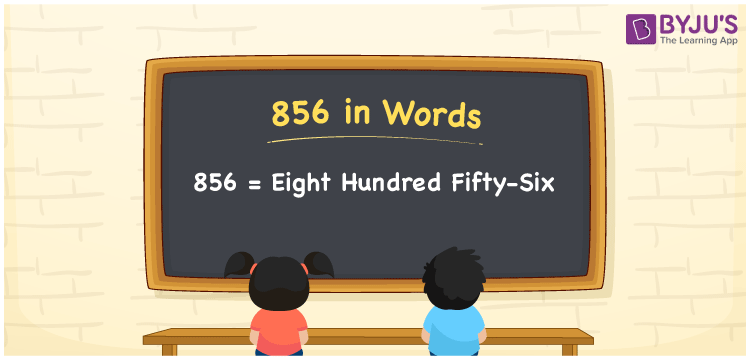# 856 in Words

856 in words is written as Eight hundred fifty-six. In both the International System of Numerals and the Indian System of Numerals, 856 is written as Eight hundred fifty-six. The number 856 is a Cardinal Number as it represents some quantity. For example, “the box weighs 856 grams”.

 856 in Words Eight hundred fifty-six Eight hundred fifty-six in Number 856

## 856 in English Words

We write 856 in English Words using the letters of the English alphabet. Therefore, we read 856 in English as “Eight hundred fifty-six.”## How to Write 856 in Words?

To write 856 in words, we shall use the place value chart. In the place value chart, write 8 in the hundreds, 5 in the tens, and 6 in the ones, respectively. Now let us make a place value chart to write the number 856 in words.

 Hundreds Tens Ones 8 5 6

Thus, we can write the expanded form as

8 × Hundred + 5 × Ten + 6 × One

= 8 × 100 + 5 × 10 + 6 × 1

= 800 + 50 + 6

= 856

= Eight hundred fifty-six.

856 is a natural number, the successor of 855 and the predecessor of 857.

856 in words – Eight hundred fifty-six

• Is 856 an odd number? – No
• Is 856 an even number? – Yes
• Is 856 a perfect square number? – No
• Is 856 a perfect cube number? – No
• Is 856 a prime number? – No
• Is 856 a composite number? – Yes

## Frequently Asked Questions on 856 in Words

Q1

### How to write 856 in words?

856 in words is written as Eight hundred fifty-six.
Q2

### How to write 856 in the International and Indian System of Numerals?

In both, the system of numerals, 856 in words, is written as Eight hundred fifty-six.
Q3

### What is the preceding number of 856?

The number that precedes 856 is 855.